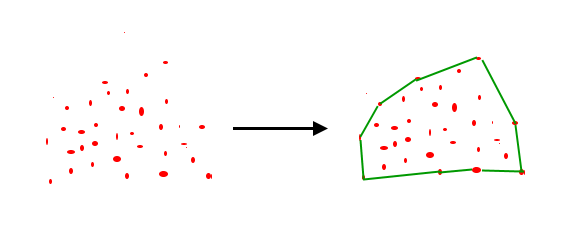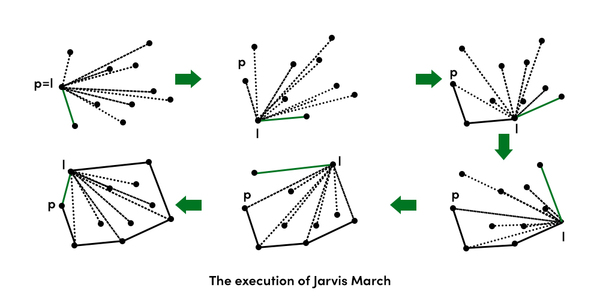# Convex Hull | Set 1 (Jarvis’s Algorithm or Wrapping)

• Difficulty Level : Hard
• Last Updated : 13 Sep, 2021

Given a set of points in the plane. the convex hull of the set is the smallest convex polygon that contains all the points of it.Attention reader! Don’t stop learning now. Get hold of all the important DSA concepts with the DSA Self Paced Course at a student-friendly price and become industry ready.  To complete your preparation from learning a language to DS Algo and many more,  please refer Complete Interview Preparation Course.

In case you wish to attend live classes with experts, please refer DSA Live Classes for Working Professionals and Competitive Programming Live for Students.

We strongly recommend to see the following post first.
How to check if two given line segments intersect?
The idea of Jarvis’s Algorithm is simple, we start from the leftmost point (or point with minimum x coordinate value) and we keep wrapping points in counterclockwise direction. The big question is, given a point p as current point, how to find the next point in output? The idea is to use orientation() here. Next point is selected as the point that beats all other points at counterclockwise orientation, i.e., next point is q if for any other point r, we have “orientation(p, q, r) = counterclockwise”. Following is the detailed algorithm.
1) Initialize p as leftmost point.
2) Do following while we don’t come back to the first (or leftmost) point.
…..a) The next point q is the point such that the triplet (p, q, r) is counterclockwise for any other point r. To find this, we simply initialize q as next point, then we traverse through all points. For any point i, if i is more counterclockwise, i.e., orientation(p, i, q) is counterclockwise, then we update q as i. Our final value of q is going to be the most counterclockwise point.
…..b) next[p] = q (Store q as next of p in the output convex hull).
…..c) p = q (Set p as q for next iteration).Below is the implementation of above algorithm.

## C++

 `// A C++ program to find convex hull of a set of points. Refer``// https://www.geeksforgeeks.org/orientation-3-ordered-points/``// for explanation of orientation()``#include ``using` `namespace` `std;` `struct` `Point``{``    ``int` `x, y;``};` `// To find orientation of ordered triplet (p, q, r).``// The function returns following values``// 0 --> p, q and r are collinear``// 1 --> Clockwise``// 2 --> Counterclockwise``int` `orientation(Point p, Point q, Point r)``{``    ``int` `val = (q.y - p.y) * (r.x - q.x) -``              ``(q.x - p.x) * (r.y - q.y);` `    ``if` `(val == 0) ``return` `0;  ``// collinear``    ``return` `(val > 0)? 1: 2; ``// clock or counterclock wise``}` `// Prints convex hull of a set of n points.``void` `convexHull(Point points[], ``int` `n)``{``    ``// There must be at least 3 points``    ``if` `(n < 3) ``return``;` `    ``// Initialize Result``    ``vector hull;` `    ``// Find the leftmost point``    ``int` `l = 0;``    ``for` `(``int` `i = 1; i < n; i++)``        ``if` `(points[i].x < points[l].x)``            ``l = i;` `    ``// Start from leftmost point, keep moving counterclockwise``    ``// until reach the start point again.  This loop runs O(h)``    ``// times where h is number of points in result or output.``    ``int` `p = l, q;``    ``do``    ``{``        ``// Add current point to result``        ``hull.push_back(points[p]);` `        ``// Search for a point 'q' such that orientation(p, q,``        ``// x) is counterclockwise for all points 'x'. The idea``        ``// is to keep track of last visited most counterclock-``        ``// wise point in q. If any point 'i' is more counterclock-``        ``// wise than q, then update q.``        ``q = (p+1)%n;``        ``for` `(``int` `i = 0; i < n; i++)``        ``{``           ``// If i is more counterclockwise than current q, then``           ``// update q``           ``if` `(orientation(points[p], points[i], points[q]) == 2)``               ``q = i;``        ``}` `        ``// Now q is the most counterclockwise with respect to p``        ``// Set p as q for next iteration, so that q is added to``        ``// result 'hull'``        ``p = q;` `    ``} ``while` `(p != l);  ``// While we don't come to first point` `    ``// Print Result``    ``for` `(``int` `i = 0; i < hull.size(); i++)``        ``cout << ``"("` `<< hull[i].x << ``", "``              ``<< hull[i].y << ``")\n"``;``}` `// Driver program to test above functions``int` `main()``{``    ``Point points[] = {{0, 3}, {2, 2}, {1, 1}, {2, 1},``                      ``{3, 0}, {0, 0}, {3, 3}};``    ``int` `n = ``sizeof``(points)/``sizeof``(points);``    ``convexHull(points, n);``    ``return` `0;``}`

## Java

 `// Java program to find convex hull of a set of points. Refer``// https://www.geeksforgeeks.org/orientation-3-ordered-points/``// for explanation of orientation()``import` `java.util.*;` `class` `Point``{``    ``int` `x, y;``    ``Point(``int` `x, ``int` `y){``        ``this``.x=x;``        ``this``.y=y;``    ``}``}` `class` `GFG {``    ` `    ``// To find orientation of ordered triplet (p, q, r).``    ``// The function returns following values``    ``// 0 --> p, q and r are collinear``    ``// 1 --> Clockwise``    ``// 2 --> Counterclockwise``    ``public` `static` `int` `orientation(Point p, Point q, Point r)``    ``{``        ``int` `val = (q.y - p.y) * (r.x - q.x) -``                  ``(q.x - p.x) * (r.y - q.y);``     ` `        ``if` `(val == ``0``) ``return` `0``;  ``// collinear``        ``return` `(val > ``0``)? ``1``: ``2``; ``// clock or counterclock wise``    ``}``    ` `    ``// Prints convex hull of a set of n points.``    ``public` `static` `void` `convexHull(Point points[], ``int` `n)``    ``{``        ``// There must be at least 3 points``        ``if` `(n < ``3``) ``return``;``     ` `        ``// Initialize Result``        ``Vector hull = ``new` `Vector();``     ` `        ``// Find the leftmost point``        ``int` `l = ``0``;``        ``for` `(``int` `i = ``1``; i < n; i++)``            ``if` `(points[i].x < points[l].x)``                ``l = i;``     ` `        ``// Start from leftmost point, keep moving``        ``// counterclockwise until reach the start point``        ``// again. This loop runs O(h) times where h is``        ``// number of points in result or output.``        ``int` `p = l, q;``        ``do``        ``{``            ``// Add current point to result``            ``hull.add(points[p]);``     ` `            ``// Search for a point 'q' such that``            ``// orientation(p, q, x) is counterclockwise``            ``// for all points 'x'. The idea is to keep``            ``// track of last visited most counterclock-``            ``// wise point in q. If any point 'i' is more``            ``// counterclock-wise than q, then update q.``            ``q = (p + ``1``) % n;``            ` `            ``for` `(``int` `i = ``0``; i < n; i++)``            ``{``               ``// If i is more counterclockwise than``               ``// current q, then update q``               ``if` `(orientation(points[p], points[i], points[q])``                                                   ``== ``2``)``                   ``q = i;``            ``}``     ` `            ``// Now q is the most counterclockwise with``            ``// respect to p. Set p as q for next iteration,``            ``// so that q is added to result 'hull'``            ``p = q;``     ` `        ``} ``while` `(p != l);  ``// While we don't come to first``                           ``// point``     ` `        ``// Print Result``        ``for` `(Point temp : hull)``            ``System.out.println(``"("` `+ temp.x + ``", "` `+``                                ``temp.y + ``")"``);``    ``}``    ` `    ``/* Driver program to test above function */``    ``public` `static` `void` `main(String[] args)``    ``{` `        ``Point points[] = ``new` `Point[``7``];``        ``points[``0``]=``new` `Point(``0``, ``3``);``        ``points[``1``]=``new` `Point(``2``, ``3``);``        ``points[``2``]=``new` `Point(``1``, ``1``);``        ``points[``3``]=``new` `Point(``2``, ``1``);``        ``points[``4``]=``new` `Point(``3``, ``0``);``        ``points[``5``]=``new` `Point(``0``, ``0``);``        ``points[``6``]=``new` `Point(``3``, ``3``);``        ` `        ``int` `n = points.length;``        ``convexHull(points, n);``       ` `    ``}``}``  ` `// This code is contributed by Arnav Kr. Mandal.`

## Python3

 `# C# program to find convex hull of a set of points. Refer``# https://www.geeksforgeeks.org/orientation-3-ordered-points/``# for explanation of orientation()` `# point class with x, y as point``class` `Point:``    ``def` `__init__(``self``, x, y):``        ``self``.x ``=` `x``        ``self``.y ``=` `y` `def` `Left_index(points):``    ` `    ``'''``    ``Finding the left most point``    ``'''``    ``minn ``=` `0``    ``for` `i ``in` `range``(``1``,``len``(points)):``        ``if` `points[i].x < points[minn].x:``            ``minn ``=` `i``        ``elif` `points[i].x ``=``=` `points[minn].x:``            ``if` `points[i].y > points[minn].y:``                ``minn ``=` `i``    ``return` `minn` `def` `orientation(p, q, r):``    ``'''``    ``To find orientation of ordered triplet (p, q, r).``    ``The function returns following values``    ``0 --> p, q and r are collinear``    ``1 --> Clockwise``    ``2 --> Counterclockwise``    ``'''``    ``val ``=` `(q.y ``-` `p.y) ``*` `(r.x ``-` `q.x) ``-` `\``          ``(q.x ``-` `p.x) ``*` `(r.y ``-` `q.y)` `    ``if` `val ``=``=` `0``:``        ``return` `0``    ``elif` `val > ``0``:``        ``return` `1``    ``else``:``        ``return` `2` `def` `convexHull(points, n):``    ` `    ``# There must be at least 3 points``    ``if` `n < ``3``:``        ``return` `    ``# Find the leftmost point``    ``l ``=` `Left_index(points)` `    ``hull ``=` `[]``    ` `    ``'''``    ``Start from leftmost point, keep moving counterclockwise``    ``until reach the start point again. This loop runs O(h)``    ``times where h is number of points in result or output.``    ``'''``    ``p ``=` `l``    ``q ``=` `0``    ``while``(``True``):``        ` `        ``# Add current point to result``        ``hull.append(p)` `        ``'''``        ``Search for a point 'q' such that orientation(p, q,``        ``x) is counterclockwise for all points 'x'. The idea``        ``is to keep track of last visited most counterclock-``        ``wise point in q. If any point 'i' is more counterclock-``        ``wise than q, then update q.``        ``'''``        ``q ``=` `(p ``+` `1``) ``%` `n` `        ``for` `i ``in` `range``(n):``            ` `            ``# If i is more counterclockwise``            ``# than current q, then update q``            ``if``(orientation(points[p],``                           ``points[i], points[q]) ``=``=` `2``):``                ``q ``=` `i` `        ``'''``        ``Now q is the most counterclockwise with respect to p``        ``Set p as q for next iteration, so that q is added to``        ``result 'hull'``        ``'''``        ``p ``=` `q` `        ``# While we don't come to first point``        ``if``(p ``=``=` `l):``            ``break` `    ``# Print Result``    ``for` `each ``in` `hull:``        ``print``(points[each].x, points[each].y)` `# Driver Code``points ``=` `[]``points.append(Point(``0``, ``3``))``points.append(Point(``2``, ``2``))``points.append(Point(``1``, ``1``))``points.append(Point(``2``, ``1``))``points.append(Point(``3``, ``0``))``points.append(Point(``0``, ``0``))``points.append(Point(``3``, ``3``))` `convexHull(points, ``len``(points))` `# This code is contributed by``# Akarsh Somani, IIIT Kalyani`

## C#

 `// C# program to find convex hull of a set of points. Refer``// https://www.geeksforgeeks.org/orientation-3-ordered-points/``// for explanation of orientation()``using` `System;``using` `System.Collections.Generic;``    ` `public` `class` `Point``{``    ``public` `int` `x, y;``    ``public` `Point(``int` `x, ``int` `y)``    ``{``        ``this``.x = x;``        ``this``.y = y;``    ``}``}` `public` `class` `GFG``{``    ` `    ``// To find orientation of ordered triplet (p, q, r).``    ``// The function returns following values``    ``// 0 --> p, q and r are collinear``    ``// 1 --> Clockwise``    ``// 2 --> Counterclockwise``    ``public` `static` `int` `orientation(Point p, Point q, Point r)``    ``{``        ``int` `val = (q.y - p.y) * (r.x - q.x) -``                ``(q.x - p.x) * (r.y - q.y);``    ` `        ``if` `(val == 0) ``return` `0; ``// collinear``        ``return` `(val > 0)? 1: 2; ``// clock or counterclock wise``    ``}``    ` `    ``// Prints convex hull of a set of n points.``    ``public` `static` `void` `convexHull(Point []points, ``int` `n)``    ``{``        ``// There must be at least 3 points``        ``if` `(n < 3) ``return``;``    ` `        ``// Initialize Result``        ``List hull = ``new` `List();``    ` `        ``// Find the leftmost point``        ``int` `l = 0;``        ``for` `(``int` `i = 1; i < n; i++)``            ``if` `(points[i].x < points[l].x)``                ``l = i;``    ` `        ``// Start from leftmost point, keep moving``        ``// counterclockwise until reach the start point``        ``// again. This loop runs O(h) times where h is``        ``// number of points in result or output.``        ``int` `p = l, q;``        ``do``        ``{``            ``// Add current point to result``            ``hull.Add(points[p]);``    ` `            ``// Search for a point 'q' such that``            ``// orientation(p, q, x) is counterclockwise``            ``// for all points 'x'. The idea is to keep``            ``// track of last visited most counterclock-``            ``// wise point in q. If any point 'i' is more``            ``// counterclock-wise than q, then update q.``            ``q = (p + 1) % n;``            ` `            ``for` `(``int` `i = 0; i < n; i++)``            ``{``            ``// If i is more counterclockwise than``            ``// current q, then update q``            ``if` `(orientation(points[p], points[i], points[q])``                                                ``== 2)``                ``q = i;``            ``}``    ` `            ``// Now q is the most counterclockwise with``            ``// respect to p. Set p as q for next iteration,``            ``// so that q is added to result 'hull'``            ``p = q;``    ` `        ``} ``while` `(p != l); ``// While we don't come to first``                        ``// point``    ` `        ``// Print Result``        ``foreach` `(Point temp ``in` `hull)``            ``Console.WriteLine(``"("` `+ temp.x + ``", "` `+``                                ``temp.y + ``")"``);``    ``}``    ` `    ``/* Driver code */``    ``public` `static` `void` `Main(String[] args)``    ``{` `        ``Point []points = ``new` `Point;``        ``points=``new` `Point(0, 3);``        ``points=``new` `Point(2, 3);``        ``points=``new` `Point(1, 1);``        ``points=``new` `Point(2, 1);``        ``points=``new` `Point(3, 0);``        ``points=``new` `Point(0, 0);``        ``points=``new` `Point(3, 3);``        ` `        ``int` `n = points.Length;``        ``convexHull(points, n);``        ` `    ``}``}` `// This code is contributed by Princi Singh`

## Javascript

 ``

Output: The output is points of the convex hull.

```(0, 3)
(0, 0)
(3, 0)
(3, 3)```

Note : The above code may produce different results for different order of inputs when there are collinear points in the convex hull. For example, it produces output as (0, 3) (0, 0) (3, 0) (3, 3) for input (0, 3), (0, 0), (0, 1), (3, 0), (3, 3) and output as (0, 3) (0, 1) (0, 0) (3, 0) (3, 3) for input as (0, 3), (0, 1), (0, 0), (3, 0), (3, 3), We generally need the farthest next point in case of collinear, we can get the desired result in case of collinear points by adding one more if condition. Please refer this modified code.
Time Complexity: For every point on the hull we examine all the other points to determine the next point. Time complexity is ?(m * n) where n is number of input points and m is number of output or hull points (m <= n). In worst case, time complexity is O(n 2). The worst case occurs when all the points are on the hull (m = n)
Set 2- Convex Hull (Graham  Scan)
Sources:
http://www.cs.uiuc.edu/~jeffe/teaching/373/notes/x05-convexhull.pdf
http://www.dcs.gla.ac.uk/~pat/52233/slides/Hull1x1.pdf